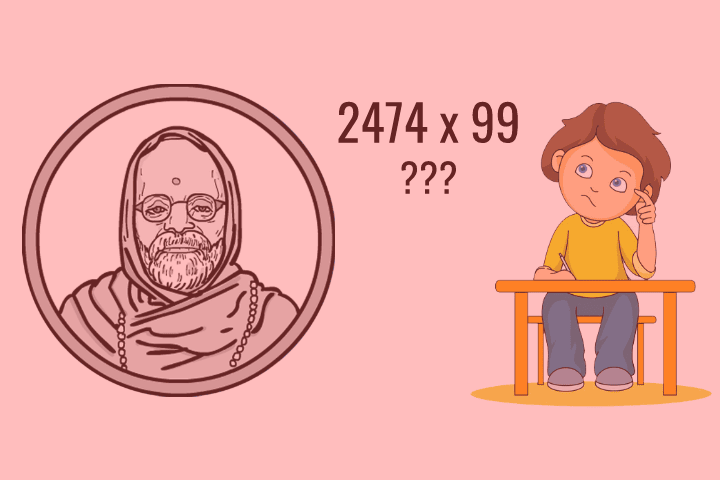Can you answer 2474 x 99 within seconds?

Can you find the square of 256 without using a calculator within seconds?

Yes, it's pretty difficult to solve these above questions without a calculator within a few seconds but, by using the Vedic Math Tricks explained in this blog post would actually help you do that easily.

For your information, the book Vedic Maths was written by an Indian monk Swami Bharati Krishna Tirtha in 1965. The book talks about various mental calculation techniques to solve mathematical problems without even using a calculator.

Must Read: 4 Ultimate Active Recall Strategies for Students

So, let's explore 5 such Vedic Math Tricks to do calculations faster than ever.Top 5 Vedic Mathematics Tricks

Here, in this blog post, I will be including the Top 5 most popular and most useful Vedic Mathematics Tricks and explain them in details.

Trick 1: Finding the Square of Any Number

Here, we will be finding out the square of 99 by using the Vedic Tricks...

Let me break the complete procedure in small-small steps:

Step 1: Choose a base closer to the given number

Here, we will choose 100 as the base (because it is closest to the 99)

Step 2: Find the difference between the given number and the base

In our case, the difference between the number and the base would be minus one i.e. -1 (99 - 100)

Step 3: Add the difference with the given number

Here, it would be 99 + (-1) = 98

Step 4: Multiply the result with the base

And, in our case, it would be 98 * 100 = 9800

Step 5: Add the multiplication of the square of the difference with the previous result

So, it would be 9800 + (-1)2 = 9800 + 1 = 9801

Hence, 992 will be equal to 9801.

Trick 2: Multiplication of Any 2 Digit Number with 11

This trick makes the multiplication of any 2 digit number with 11 very easy. You will be able to solve these types of questions within 1-2 seconds after learning this trick.

Example 1: 36 x 11

Here, you will have to keep both digits as it is and in the middle of the digits simply write their Sum.

In this case, it would be: 3 (3 + 6) 6 = 396

Note: Please note that here you don't have to multiply the digits.

Let's take another example here,

Example 2: 53 x 11

And, as you would have already guessed, it will be 5 (5 + 3) 3 = 583

But, it becomes a little different when the sum of the 2 digits is greater than 9. Let's see it by another example.

Example 3: 99 x 11

In this case, all the processes will be almost same as the previous one except you will have to write only the last digit of the sum of both digits in the middle and add 1 to the very first digit of the result.

Here's how,

9 (9 + 9) 9 = 9 (18) 9 = (9 + 1) 8 9 = 1089

Let's make it more clear with 1 more example,

Example 4: 67 x 11

6 (6 + 7) 7 = 6 (13) 7 = (6 + 1) 3 7 = 737

Isn't it awesome?

Trick 3: Division of Large Number by 5

However, dividing any number which is divisible by 5 with 5 is already easy, but in this trick, I will show you how to divide any 5 digits number with 5, within seconds.

Let me break this into small steps.

Example 1: 3132 / 5

Step 1: Multiply the number with 2

In our case, it would be 3132 x 2 = 6264

Step 2: Move the decimal to the left by 1

Here, 626.4

Let's try another question.

Example 2: 5491 / 5

First, multiply by 2: 5491 * 2 = 10982

So, 5491 / 5 = 1098.2

Isn't it simple?

Trick 4: Multiplication of Any Two 2 Digit Numbers (from 11 to 19)

How much time will it take you to multiply 12 x 18?

Quite long, right?

Here comes the trick.

Step 1: Add the unit digit of the smaller number to the larger number

Here, in our case, 18 + 2 = 20

20 x 10 = 200

2 x 8 = 16

Step 4: Add both numbers (which you obtained in Step 2 and Step 3)

200 + 16 = 216

So, 12 x 18 = 216

Trick 5: Multiply Any Number with 5

This is the best trick to multiply any large or small number with 5 just within seconds. The trick works little different for the even and odd numbers.

First, we will see the Even Numbers

Multiplication of Even Numbers by 5

Just divide the number by 2 and then write 0 at the end of it. Let me show it to you.

Example 1: 1662 x 5

First, 1662 / 2 = 831

Easy peasy, right?

Example 2: 1952364 x 5

1952364 / 2 = 976182

So, 1952364 * 5 = 9761820

Multiplication of Odd Numbers by 5

In the case of Odd Numbers, you will have to subtract 1 from the number before dividing with the 2, and, after that write 5 to the end of the number.

Here's how,

Example 1: 1637 x 5

First, (1637 - 1) / 2 = 1636 / 2 = 818

Now, write 5 at the end i.e. 8185

So, 1637 * 5 = 8185

That's it.Final Words

You just saw how useful are Vedic Mathematics Tricks when it comes to the complicated Mathematical calculations without using any calculator.

It is almost impossible to mention all those tricks here in this blog post. But, you can always go for the Vedic Mathematics book on Amazon. I have linked the book below:

5 Amazing Vedic Math Tricks to Calculate 10x FasterReviewed by Deepak on Friday, May 31, 2019 Rating: 5